# 表连接查询 条件在On与Where后区别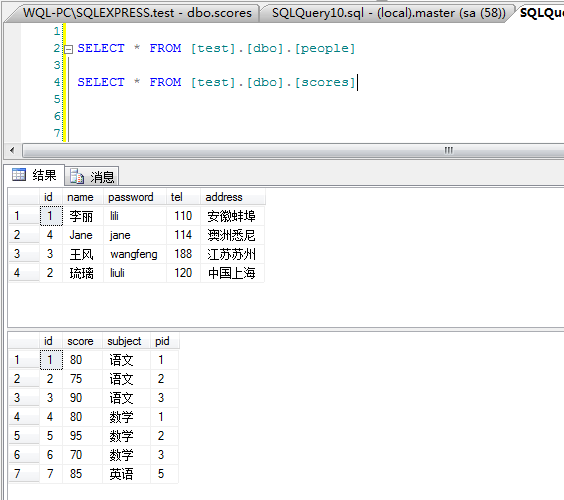1. LEFT JOIN 测试
1.1条件在On后：

  SELECT
s.*,
p.*
FROM [test].[dbo].[scores] s
Left JOIN [test].[dbo].[people] p ON 1=1 AND s.pid=p.id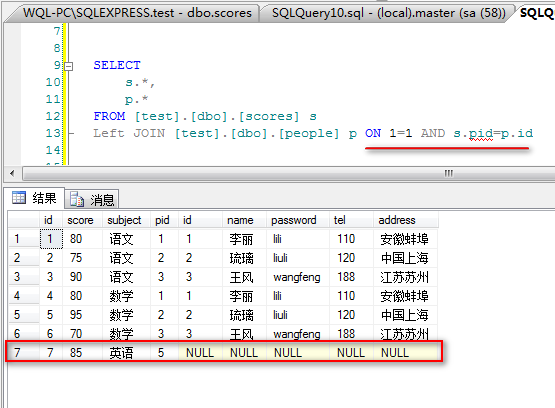1.2条件在Where后：

  SELECT
s.*,
p.*
FROM [test].[dbo].[scores] s
Left JOIN [test].[dbo].[people] p ON 1=1
Where s.pid=p.id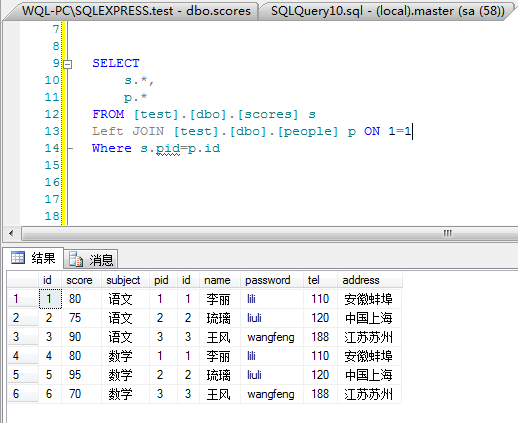1.3 区别：
可以看到：表连接条件放在Where后面，LEFT JOIN的作用失效。

2. Right JOIN 测试
2.1条件在On后：

  SELECT
s.*,
p.*
FROM [test].[dbo].[scores] s
Right JOIN [test].[dbo].[people] p ON 1=1 AND s.pid=p.id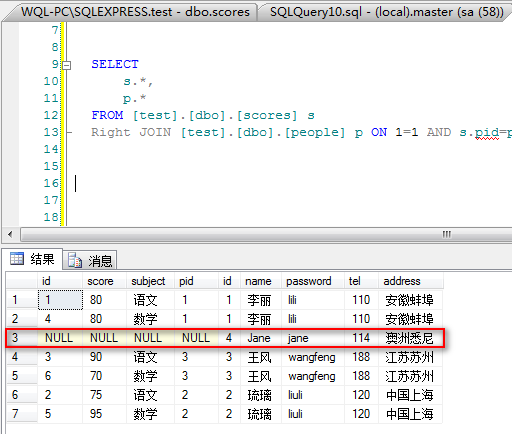2.2条件在Where后：

  SELECT
s.*,
p.*
FROM [test].[dbo].[scores] s
Right JOIN [test].[dbo].[people] p ON 1=1
Where s.pid=p.id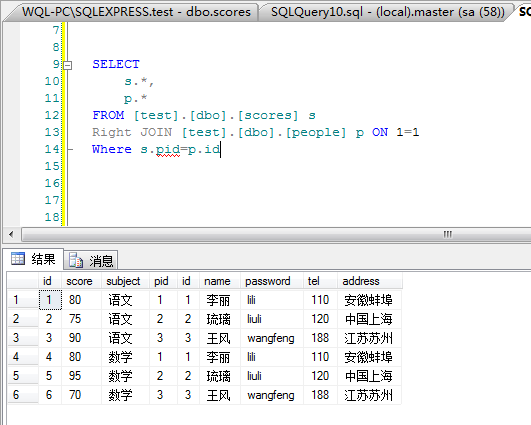2.3 区别：
可以看到：表连接条件放在Where后面，Right JOIN的作用失效。

3.总结：
所有的连接条件都必需要放在ON后面，不然前面的所有LEFT,和RIGHT关联将作为摆设，而不起任何作用。